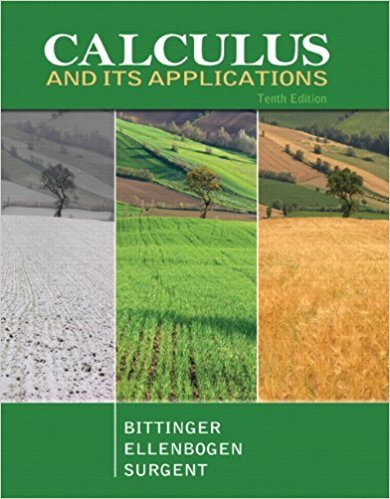×
×

# Solutions for Chapter 6.1: Functions of Several Variables## Full solutions for Calculus and Its Applications | 10th Edition

ISBN: 9780321694331Solutions for Chapter 6.1: Functions of Several Variables

Solutions for Chapter 6.1
4 5 0 287 Reviews
31
0
##### ISBN: 9780321694331

This expansive textbook survival guide covers the following chapters and their solutions. Calculus and Its Applications was written by and is associated to the ISBN: 9780321694331. Chapter 6.1: Functions of Several Variables includes 38 full step-by-step solutions. Since 38 problems in chapter 6.1: Functions of Several Variables have been answered, more than 24272 students have viewed full step-by-step solutions from this chapter. This textbook survival guide was created for the textbook: Calculus and Its Applications, edition: 10.

Key Calculus Terms and definitions covered in this textbook
• Chord of a conic

A line segment with endpoints on the conic

• Demand curve

p = g(x), where x represents demand and p represents price

• Frequency

Reciprocal of the period of a sinusoid.

• Gaussian curve

See Normal curve.

• Higher-degree polynomial function

A polynomial function whose degree is ? 3

• Identity function

The function ƒ(x) = x.

• Index of summation

See Summation notation.

• Leibniz notation

The notation dy/dx for the derivative of ƒ.

• Linear function

A function that can be written in the form ƒ(x) = mx + b, where and b are real numbers

• Logistic regression

A procedure for fitting a logistic curve to a set of data

• Lower bound test for real zeros

A test for finding a lower bound for the real zeros of a polynomial

• Measure of an angle

The number of degrees or radians in an angle

• Range of a function

The set of all output values corresponding to elements in the domain.

• Reciprocal identity

An identity that equates a trigonometric function with the reciprocal of another trigonometricfunction.

• Right triangle

A triangle with a 90° angle.

• Stem

The initial digit or digits of a number in a stemplot.

• Transverse axis

The line segment whose endpoints are the vertices of a hyperbola.

• Triangular number

A number that is a sum of the arithmetic series 1 + 2 + 3 + ... + n for some natural number n.

• Unbounded interval

An interval that extends to -? or ? (or both).

• Zero factorial

See n factorial.

×